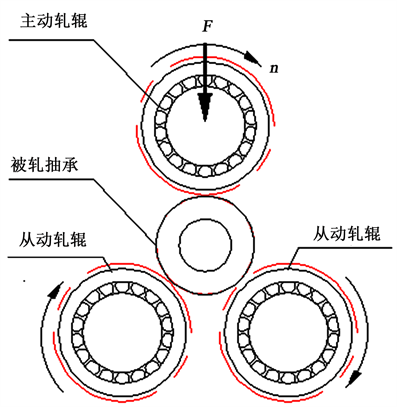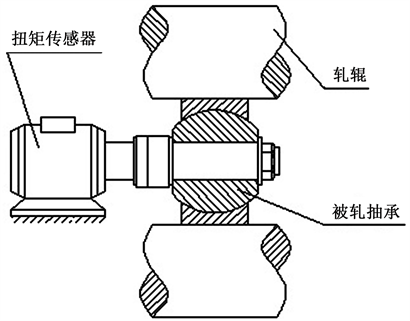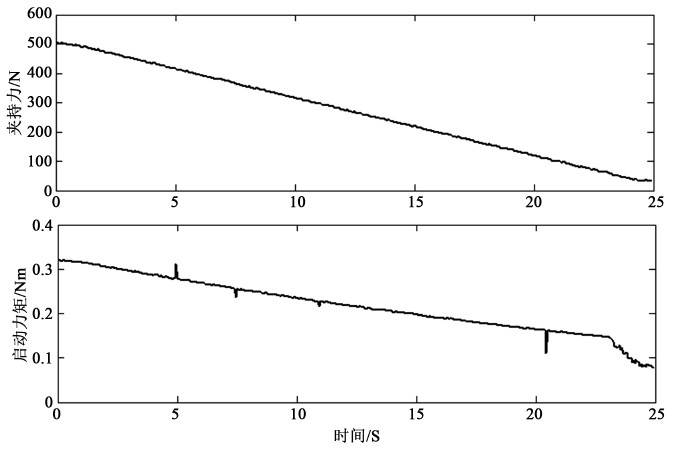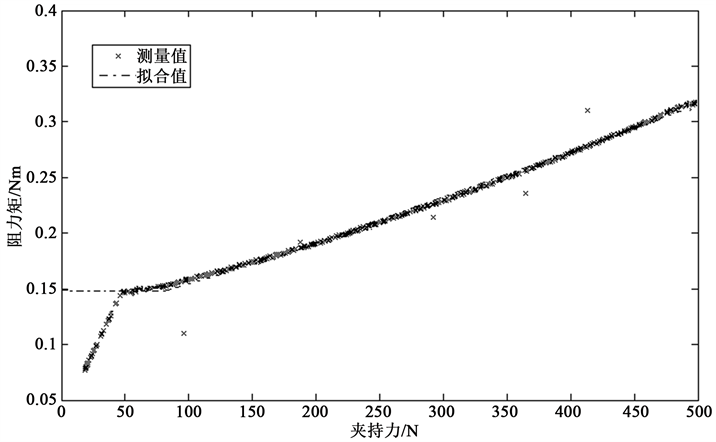﻿ 自润滑关节轴承游隙调节工序中的无载阻力矩在线测试技术 An Approach to Measuring No-Load Rotational Torque of Self-Lubricating Spherical Bearing in Rolling Clearance Pro-cess

Mechanical Engineering and Technology
Vol.07 No.03(2018), Article ID:25664,6 pages
10.12677/MET.2018.73025

An Approach to Measuring No-Load Rotational Torque of Self-Lubricating Spherical Bearing in Rolling Clearance Process

Hongyu Liu1,2

1Shanghai Bearing Technology Research Institute, Shanghai

2Shanghai Special Bearing Engineering Technology Center, ShanghaiReceived: Jun. 1st, 2018; accepted: Jun. 21st, 2018; published: Jun. 28th, 2018ABSTRACT

Rolling clearance is a type of means of adjusting the no-load rotational breakaway torque of a self-lubricating spherical bearing. Based on the bilinear model of rotational torque vs. rolling force in the rolling process, a method to evaluating the no-load torque is present, by detecting the variations in the rotational torque with the rolling force during the uploading or unloading stage in the process. The method proposed in this paper helps to improve the accuracy of rolling clearance of self-lubricating spherical bearings.

Keywords:Self-Lubricating Spherical Bearing, No-Load Rotational Breakaway Torque, On-Line Measure

1上海市轴承技术研究所，上海

2上海特种轴承工程技术中心，上海Copyright © 2018 by author and Hans Publishers Inc.

This work is licensed under the Creative Commons Attribution International License (CC BY).

http://creativecommons.org/licenses/by/4.0/1. 引言

2. 自润滑关节轴承游隙调节工序中的无载阻力矩在线检测原理Figure 1. The clearance process of three-roll-type rolling self-lubricating spherical bearingFigure 2. The measurement scheme of bearing resistance moment

2.1. 三辊夹持下的阻力矩模型

${T}_{s}=\left\{\begin{array}{ll}{T}_{\text{s0}}+\alpha \left({F}_{\text{fix}}-{F}_{\text{fix0}}\right)\hfill & {F}_{\text{fix}}\ge {F}_{\text{fix0}}\hfill \\ {T}_{\text{s0}}\hfill & {F}_{\text{fix}}<{F}_{\text{fix0}}\hfill \end{array}$ (1)

${T}_{\text{s}}<\rho {F}_{\text{f}}$ (2)

2.2. 三辊夹持下无载启动力矩的检测

1) 轴承外圈和轧辊的摩擦半径的评估

a) 根据轧辊和轴承外圈材料，初定轴承外圈摩擦半径 $\rho ={\rho }^{\left(0\right)}$ ，从原始数据中选取满足 $\frac{{T}_{\text{s}j}}{{F}_{\text{fix}j}}>{\rho }^{\left(0\right)}$ 的K对数据对(Tsj，Ffixj) ( $j=\text{1},\text{2},\cdots ,K$ )。

b) 计算阻力矩和夹紧力之比的均值和均方差

${\rho }^{\left(1\right)}=\frac{1}{K}\sum _{j=1}^{K}\frac{{T}_{\text{s}j}}{{F}_{\text{fix}j}}$ (3)

${\sigma }^{\left(1\right)}=\sqrt{\frac{1}{K}\sum _{j=1}^{K}{\left(\frac{{T}_{\text{s}j}}{{F}_{\text{fix}j}}-{\rho }^{\left(1\right)}\right)}^{2}}$ (4)

c) 若误差 ${\sigma }^{\left(1\right)}$ 超过允差，剔除其中的超差的数据对，转(b)；否则，得到轴承外圈摩擦半径 $\rho ={\rho }^{\left(1\right)}$

2) 无载启动力矩的评估

a) 按Ffix降序排列数据对(Tsj，Ffixj) ( $j=\text{1},\text{2},\cdots ,M$ )。

b) 取 $1 ，对(Tsi，Ffixi) ( $i=\text{1},\text{2},\cdots ,p$ )利用最小二乘法拟合成直线 ${T}_{\text{s}i}=\alpha {F}_{\text{fix}i}+\beta$

c) 将(Tsi，Ffixi) ( $i=p+1,2,\cdots ,M$ )拟合成水平线，即

${T}_{\text{s0}}=\frac{1}{M-p}\sum _{i=p+1}^{M}{T}_{\text{s}i}$ (5)

d) 取双线段拟合误差

$E=\sum _{i=1}^{p}{\left({T}_{\text{s}i}-\alpha {F}_{\text{fix}i}-\beta \right)}^{2}+\sum _{i=p+1}^{M}{\left({T}_{\text{s}i}-{T}_{\text{s0}}\right)}^{2}$ (6)

3. 试验分析和讨论

${T}_{\text{s}}=\left\{\begin{array}{ll}0.1481+0.0004286\left({F}_{\text{fix}}-82.2\right)\hfill & {F}_{\text{fix}}\ge 82.2\hfill \\ 0.1481\hfill & {F}_{\text{fix}}<82.2\hfill \end{array}$Figure 3. The grasping force and resistant moment curves with respect to time respectively during the process of roll unloadingTable 1. The off-line and on-line measured result comparison of starting torque for GE15Figure 4. The curve of grasping force with respect to resistant moment

4. 结论

An Approach to Measuring No-Load Rotational Torque of Self-Lubricating Spherical Bearing in Rolling Clearance Pro-cess[J]. 机械工程与技术, 2018, 07(03): 200-205. https://doi.org/10.12677/MET.2018.73025

1. 1. 张令, 赵颖春. 自润滑关节轴承无载旋转启动力矩测量标准分析[J]. 润滑与密封, 2015, 40(2): 112-115.

2. 2. 林晶, 张令. 湿热对自润滑关节轴承无载旋转启动力矩的影响[J]. 轴承, 2013(8): 30-32.

3. 3. 李宝福, 刘亮. 自润滑关节轴承无载启动力矩测量方法分析[J]. 轴承, 2013(1): 81-83.

4. 4. 从志斌, 李宝福. 自润滑关节轴承摆动摩擦力矩的测量方法[J].计量与测试技术, 2016, 43(9): 43-46.

5. 5. 刘红宇, 李宝福. 自润滑关节轴承力矩在线即时检测与控制系统及方法[P]. 中国专利: 201110456320.4, 2012-07-04.

6. 6. 李英, 李宝福, 等. 自润滑关节轴承接触应力分析[J]. 轴承, 2016(5): 32-36.

7. 7. 田垅, 刘宗田. 最小二乘法分段直线拟合[J]. 计算机科学, 2012, 39(6A): 482-484.